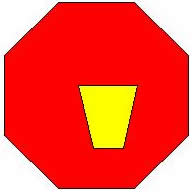#### You may also like### Modular Fractions

We only need 7 numbers for modulus (or clock) arithmetic mod 7 including working with fractions. Explore how to divide numbers and write fractions in modulus arithemtic.Find all real solutions of the equation (x^2-7x+11)^(x^2-11x+30) = 1.### Areas and Ratios

Do you have enough information to work out the area of the shaded quadrilateral?

# Proof of Pick's Theorem

##### Age 16 to 18 Challenge Level:We are allowed to assume that any polygon, convex or not, can be split into a finite number of non-overlapping triangles. However in this proof we assume that the interior of the polygon does not have any holes like the red polygon shown with a yellow hole in the diagram. Pick's formula is related to Euler's formula and ${\rm area }(P) = i + {1\over 2}p - q$ where $q$ depends on the number of holes.